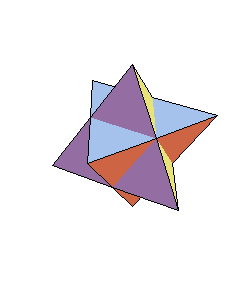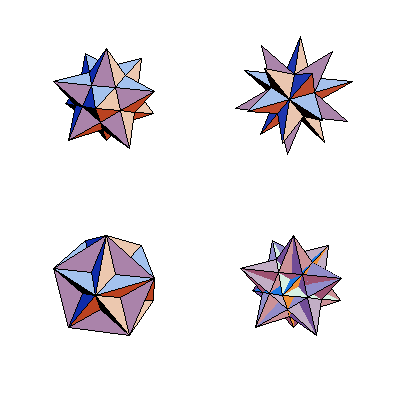## Introduction

Stellation means the extension the faces of a polyhedron until they intersect with the extensions of other faces, such that the symmetry of the original polyhedron is preserved. The set of all possible edges of the stellated forms can be obtained by finding all intersections of the plane of one face with the planes of all other faces. No stellations are possible for the tetrahedron and the cube. The only stellation of the octahedron is the stella octangula, a compound of two dual tetrahedra. The two tetrahedra are related to each other by inversion and can easily be drawn.

```  tetra = Polyhedron[Tetrahedron];
dual  = AffineShape[ tetra, {-1, -1, -1} ];```

`  Show[{tetra, dual}];`The dodecahedron admits three stellations, discovered by Kepler in 1619 (the small stellated dodecahedron and the great stellated dodecahedron), and by Poinsot in 1809 (the great dodecahedron). They are all regular (but have non-convex faces or vertices; see also [Blachmann93]). A regular polyhedron has faces that are congruent regular polygons (triangles, squares, and pentagons are the only ones that occur). All vertices show the same arrangement of faces around them. The only non-convex polygon than can occur as face of a regular (non-convex) polyhedron is the pentagram (five-pointed star).

Together with one stellation of the icosahedron---the great icosahedron---the stellated dodecahedra form all non-convex regular polyhedra. The four regular star polygons are shown here; the code to produce this graphic uses the package `Graphics`Polyhedra``. The command `Polyhedron[name]` generates a `Graphics3D` object of the named polyhedron.

```  starpoly =
Map[ Polyhedron,
{{SmallStellatedDodecahedron, GreatStellatedDodecahedron},
{GreatDodecahedron, GreatIcosahedron}
}, {2} ];```

`  Show[ GraphicsArray[starpoly] ];`Next: Stellations of the Icosahedron